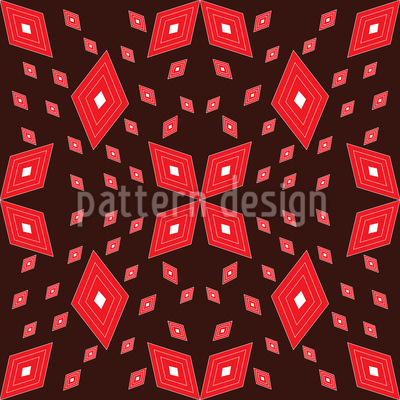••••• Change color

# Matrix Of Rhombs Seamless Vector Pattern Design

Keywords
Floral, Hot, Matrix, Mirrored, Geometric, Rhomb, Geometry, Expressive, Geometric Pattern, Geometrical, Rhombus, Rhombuses, Rhombs, Rhombic, Lozenge Matrix Of Rhombs design, Matrix Of Rhombs vector, Matrix Of Rhombs pattern, Matrix Of Rhombs all over print, Matrix Of Rhombs illustration, Matrix Of Rhombs ornament, Matrix Of Rhombs pattern design, Matrix Of Rhombs artwork, Matrix Of Rhombs vector art, Matrix Of Rhombs background pattern, Matrix Of Rhombs textile, Matrix Of Rhombs seamless, Matrix Of Rhombs repeating pattern, Matrix Of Rhombs surface
Designer Razina Athar ID #8768
Description Expressive geometrically! Argyle ornaments form this matrix.
Prices